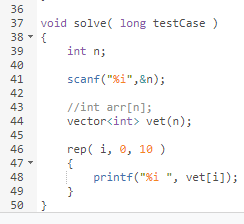# C Language: How to initialize an integer array with 0?

Hello, Guys,

To initialize an integer array I usually use a for loop, something like:

for(i=0: i<n; i++)
array[i]=0;

Like I did in this solution:
https://www.codechef.com/viewsolution/40987043

I’m wondering if there is an easier and faster way to do it. I checked online that it is possible to do “array={0}” or “array={ }”, but I tested on codechef compiler for C and it does not work.

Do you know of any other way to do it that works on codechef compiler for C?

Thanks in advance for the help.1 Like

int arr={0};
its working fine. check it

Initialising an array can be done in the following way:

``````int a;
// a will be some garbage value, but rest all will be 0's.

/*
Initialising with 0
*/

// Static way of initialisation
int a = {0}; // all elements will be 0

// Or use #define to define the size of array.
#define size 10000
int b[size] = {0}; // All elements will be 0 in this case too

// The following doesn't work
int n = 100;
int c[n] = {0};  // error: variable-sized object may not be initialized
``````
``````/*
Initialising with some other value (say 15)
*/

// The following doesn't work
int a = {15}; // a will be 15, but rest all values will be 0

// Use for loop to initialise
int a;
for(int i=0;i<100;i++)
a[i] = 15;

// Initialisation won't affect the time complexity, since O(n) loops are lightning fast in C.
``````
1 Like

I’ll just add this here, but you could use a vector, which initializes all elements to 0 by default.

``````vector<int> v(size);
``````

memset(array, 0, sizeof(array));

here all the elements of the array will be initialized to 0
you can also set the number of bytes you want to initialize (from the starting of the array)
something like this

memset(array, 0, n * sizeof(arr));

will initialize first n elements to 0

note-> you have to add the header <memory.h> or <string.h> in order to use memset function!

1 Like

Thank you suman_18733097, I tested each example and worked exactly as you mentioned. Thanks for your contribution. I think I was having problem with variable sized array.

You are correct, sourav2901. I tested and It works for a fixed sized array.

1 Like

Thanks, inclusiveor. I tried this :But I get this error:Thanks for the help anyways.Thank you for the help, eraldo_coil. It also works for variable sized arrays. That is cool. Thanks for your contribution.

I noticed that it does not work with values different than zero in the second argument of the function right? It seems to work with strings, but not with int arrays. I am trying for example:
memset(array, 10, n * sizeof(arr));

Yeah, memset cannot be used to fill an Integer array with values other than {-1,0}.
You can refer about it here.

Oh sorry, I misunderstood this discussion to be about C++. My bad.

For using vectors, include the “vector” header file:

``````#include<vector>
``````

or if you wanna be lazy about including the required header files for the particular problem statement, you can include this one header file:

``````#include<bits/stdc++.h>
``````

This header file contains all the necessary header files such as iostream, algorithm, vector, math, and many useful functions!

What I stated above regarding vectors and that other header file "bits/stdc++.h> is possible in C++.

In C though, you’ll have to use arrays!

int a[size] = {0};

works just fine, where “size” is the size of the array. I checked it in codechef C compiler.

Well, as stated earlier in this discussion,

when we try to initialise entire array elements with non-zero element in this manner, only first element will take the value. Rest all will be 0.

You should have even tested for non-zero values.

bro can you do this

@suman_18733097 ok alright! I didn’t test for non zero values, since the question up above was requiring the answer for only zero. My bad!